# 22. Visualising complex functions¶

## 22.1. One-dimensional functions¶

Consider a “normal” plot of $$\sin(t)$$:

:

import sympy
sympy.init_printing()

%matplotlib inline

:

t = sympy.Symbol('t')

:

sympy.plot(sympy.sin(t))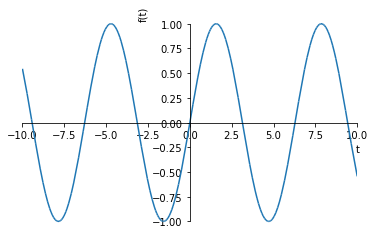:

<sympy.plotting.plot.Plot at 0x10f372470>


This allows us to see exactly what the of $$\sin(t)$$ is for every value of $$t$$. To do this, we need two dimensions: one for the value of $$t$$ and one for $$\sin(t)$$.

If we want to plot a function of two variables we start needing three dimensions. The following plot shows a “fake” 3d plot. It’s fake because in fact there are only two dimensions available on your computer screen. So we’re already in a bit of trouble when trying to visualise the relationships represented by higher dimensional functions.

:

x, y = sympy.symbols('x, y')

:

f2 = sympy.sin(x) + sympy.cos(y)

:

sympy.plotting.plot3d(f2)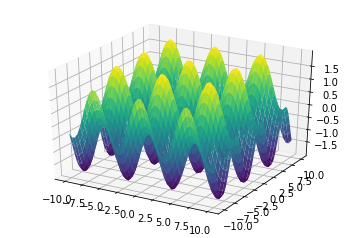:

<sympy.plotting.plot.Plot at 0x1113c2320>


We can make up for some of this lack by using colors to represent another axis.

:

import numpy
import matplotlib.pyplot as plt

:

f2numeric = sympy.lambdify((x, y), f2, 'numpy')

:

xx, yy = numpy.mgrid[-10:10:0.1, -10:10:0.1]

:

zz = f2numeric(xx, yy)

:

plt.pcolor(xx, yy, zz)

:

<matplotlib.collections.PolyCollection at 0x111922550>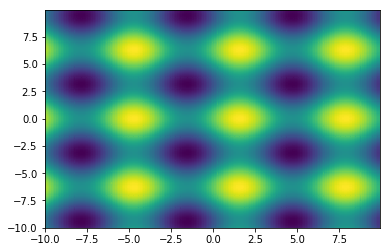For transfer functions, we have an even bigger challenge, because we have two input variables (the real and imaginary part of $$s$$) and two output variables (the real and imaginary part of $$G(s)$$). One solution to the problem is to use colors for $$\angle G(s)$$ and brightness for $$|G(s)|$$. This is known as domain colouring and is supplied by mpmath.cplot.

:

try:
import mpmath
except:
from sympy import mpmath

:

s = sympy.Symbol('s')

G = 1/(s**2 + s + 1)
Gnumeric = sympy.lambdify(s, G)
mpmath.cplot(Gnumeric)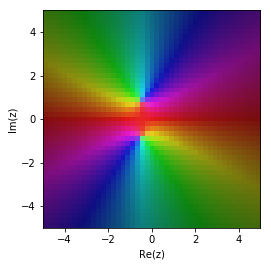We can get a smoother result by plotting with more points

:

mpmath.cplot(Gnumeric, points=10000)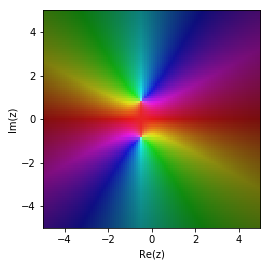The roots of the denominator appear as two bright regions.

Another solution is to plot the image of $$G(s)$$ as $$s$$ goes through a particular path. We will speak more of this when we cover the frequency domain, but have a look at this example. We generate values of $$s$$ in a circle of diameter 1 (radius 0.5) around the origin, then plot $$G(s)$$ for all these $$s$$ values. This is known as the image of $$G(s)$$ for these values of $$s$$.

:

theta = numpy.linspace(0, 2*numpy.pi)  # angles around the circle
s = 0.5*(numpy.cos(theta) + numpy.sin(theta)*1j) # The circle with radius 0.5
Gs = Gnumeric(s)

:

plt.plot(s.real, s.imag)
plt.plot(Gs.real, Gs.imag)
plt.axis('equal');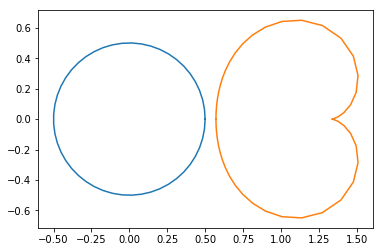[ ]: